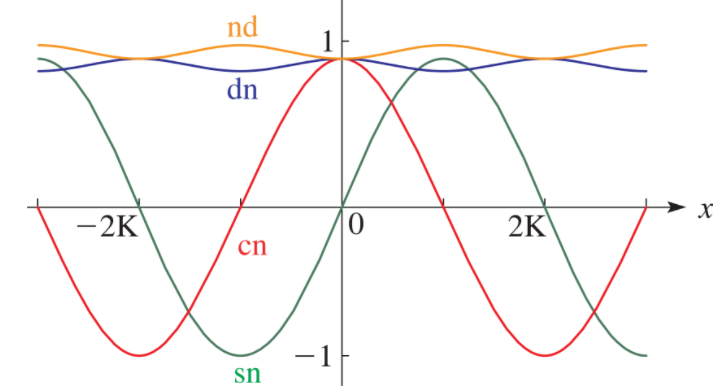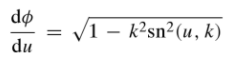# Jacobi Elliptic Functions

The Jacobi Elliptic functions are a way to express the amplitude φ in terms of an elliptic integral u and modulus k. They share many properties with trigonometric functions and can be thought of as trig function generalizations; In limiting cases where the parameter tends to zero, the Jacobi elliptic functions sn and cn reduce to their trigonometric counterparts: the sine function and cosine function.Line graphs of the Jacobi elliptic functions nd(x, k), dn (x, k), cn (x, k) and sn (x, k), illustrating near trigonometric behavior. Image: NIST.

They are named after 19th century mathematician Carl Gustav Jacob Jacobi, who contributed to the theory of elliptic functions.

## Applications of Jacobi Elliptic Functions

These functions are mostly noted for their historical importance. Practical applications for Jacobi elliptic functions include:

They also appear in various problems of classical dynamics, electrostatics, and hydrodynamics.

## Types

There are twelve types of Jacobi elliptic functions denoted by pw(u,k), where:

• u is the real-numbered or complex-numbered argument,
• k is the real or complex modulus (or modular angle, or parameter). Note that convention calls for m if you’re using the parameter or k if you’re using the modulus (which is the square root of m) or modular angle (defined as m = sin2 α),
• p and q can be any of the letters c, s, n or d.

The main types are:

• sn(u, k) = Jacobi elliptic sine function of modulus k, defined as sin φ = sin am(u, k). Analogous to the trigonometric sine function.
• cn(u, k) = Jacobi elliptic cosine function, defined as cos φ = cos am(u, k). Analogous to the trigonometric cosine function.
• tn(u, k) = Jacobi elliptic tangent function: sin φ/cos φ = sn(u, k) / cn (u, k)
• dn (u, k) = difference function (the derivative of φ) =The functions dc, nc, and dc are extensions to imaginary arguments.

## Properties of Jacobi Elliptic Functions

Many of these properties follow from properties of trigonometric functions (Lutovac et al., 2001):

• sn2(u, k) + cn(u, k) = 1
• k2sn2(u, k) + dn2(u, k) = 1
• sn(u, k) = sn(-u, k)
• cn(u, k) = cn(-u, k)
• sn(0, k) = 0
• sn(K, k) = 1
• cn(0, k) = 1
• cn(K, k) = 0

## References

Lutovac, M. et al., (2001). Filter Design for Signal Processing Using MATLAB and Mathematica. Prentice Hall.
NIST. Digital Library of Mathematical Functions. Retrieved December 3, 2020 from: https://dlmf.nist.gov/22.3#F1

CITE THIS AS:
Stephanie Glen. "Jacobi Elliptic Functions" From StatisticsHowTo.com: Elementary Statistics for the rest of us! https://www.statisticshowto.com/jacobi-elliptic-functions/